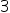Maths-
General
Easy

Question

# Identify the non statistical question :

## tell me about fractionsno. of sides of a triangle brief introduction of quadrilateraldefine fractionHint:

## The correct answer is: no. of sides of a triangle

### A non-statistical question is a question which doesn't give a variety of answers.Number of sides of a triangle are fixed and are equal towhich cannot be varied.So, number of sides of a triangle is a non-statistical question.#### With Turito Foundation.#### Get an Expert Advice From Turito.